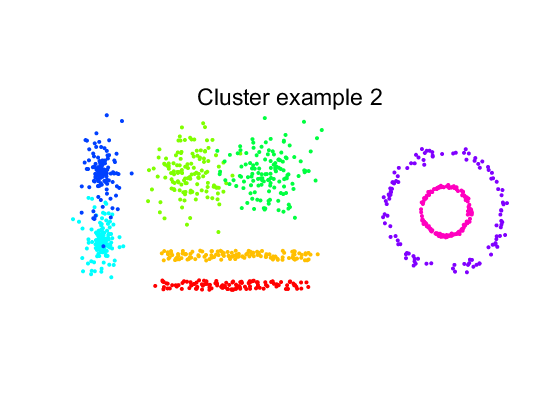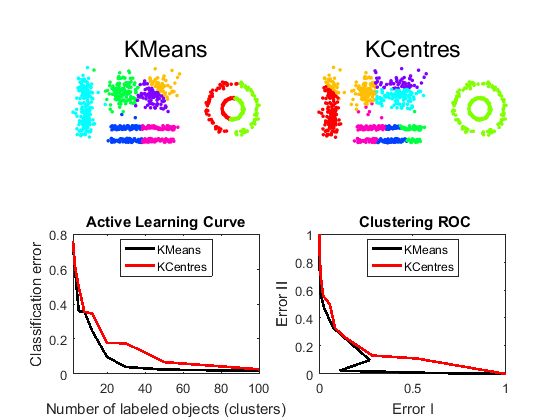# ct_kmeans Centroid-based clustering

PRTools and ClusterTools should be in the path.

Goto ClusterTools examples for a review of all examples.

## Prepare environment

```prtime(10)                   % restrict iterative optimisation to 10s
delfigs                      % delete existing figures
randreset      ;             % takes care of reproducability
prwarning(2)                 % show warnings
```

## Define a dataset

we use some standard routines to create 8 two-dimensional clusters. They are labeld to compare cluster results with the desired labeling.A

```m = 1000;
a = gendatclust2(m);
scattern(a); axis equal
title(getname(a));
```## Run two clustering procedures after removing the label information.

```x = +a; % remove labels
K = [2 3 5 8 12 20 30 50 100]; % desired cluster sizes
procs = {'KMeans','KCentres'};
err_I = cell(1,2);
err_II= cell(1,2);
figure;
for i=1:2
subplot(2,2,i);
lab = x*clustk(K,procs{i});  % execute KMeans or KCentres
scatn(lab(:,4),x,procs{i});  % scatterplot
markcols(1);                 % show distinguishable colors colors
axis equal;
err_I{i}  = clusteval(lab,a,'actl');
err_II{i} = clusteval(lab,a,'roc');
end

subplot(2,2,3); h1 = plote(err_I,10,'legend',procs);
subplot(2,2,4); h2 = plote(err_II,10,'legend',procs);
```